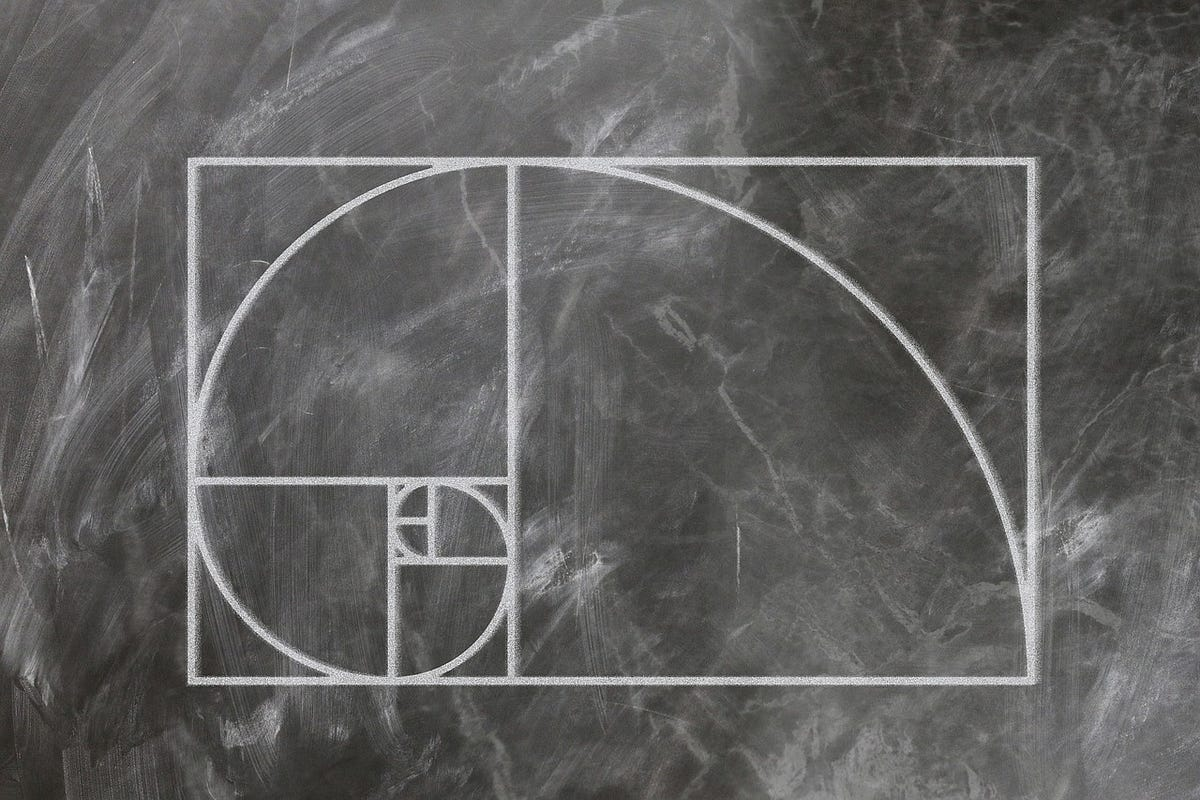# Improving the performance of the recursive Fibonacci implementation using closuresIn this article, we will develop a solution in Javascript using recursion, and we will improve its performance using closures.

If you ever encountered yourself doing some practice on recursion, I’m quite sure you faced the Fibonacci sequence algorithm. If you are not familiar with this problem, take a look at what Wikipedia has to say about it:

In mathematics, the _**_Fibonacci numbers_, commonly denoted Fn, form a sequence called the _Fibonacci sequence**, such that each number is the sum of the two preceding ones.

So, if we start with 0 and 1, this is how the first N Fibonacci numbers look like:

``0, 1, 1, 2, 3, 5, 8, 13, 21, 34, ...``

As you can see, each number (apart from the first two) is derived from the sum of the previous 2. For example, 13 **is the result of adding 8 and 5**.

In this article, we will develop a solution in Javascript using recursion, and we will improve its performance using closures.

## First solution

Let’s try to code a function that will receive a number n, and will retrieve the Fibonacci number at the n position. We will use the recursive approach:

``````function fibonacci(n) {
if (n <= 0) {
return 0;
} else if (n <= 2) {
return 1;
}
return fibonacci(n - 1) + fibonacci(n - 2);
}``````

(Note that some theories omit the first 0 from the Fibonacci sequence, in which case all you have to do is to omit the first if statement and change the second one to be n < 2 )

## Javascript Closure: How to Use Closure in JavaScript

Javascript Closure is the aggregate of functions clumped together with the references to its surrounding environment.

## A practical example of Javascript closures

Closures are something that many companies would like you to know before working for them, therefore, Most developers today, know about closures in Javascript. Don’t be sad if you don’t, it’s not something you generally use on your every-day work (well, you may, but it’s not so common).

## While You Don't Understand Recursion, Read Recursion: by Randy Taylor

Recursion is the one idea I constantly use while I solve coding problems. Most of the time I don’t start by thinking “RECURSION WILL SOLVE THIS!”. However recursion just ends up being the logical way to reach an answer.

## JavaScript Recursion and Memoization for Dummies

Now, I know I am not a dummy, and I’m gonna assume you are not a dummy either. However, we all have different learning styles, and sometimes it takes a while for a new concept to “click”, and it can often make you wonder if you’re a dummy until it does. Or maybe that’s just me. Recursion is one of those concepts that I used to find myself relearning every time I didn’t use it for a while. I remembered generally what it is and why we need it, but after a long lay off, programming recursively was always a struggle.

## Hire Dedicated JavaScript Developers -Hire JavaScript Developers

Hire dedicated JavaScript Developers who are proficient in AngularJS, ReactJS, NodeJS, & VueJS frameworks. Get flexible hiring models as per your business requirements.